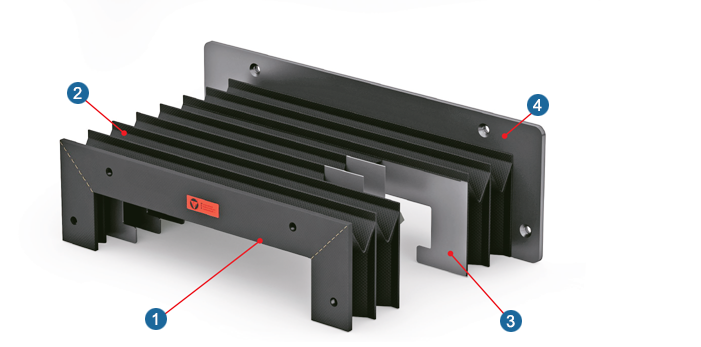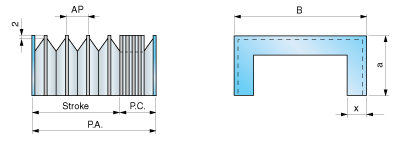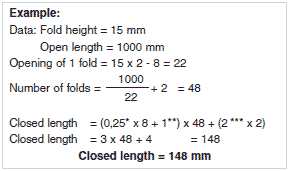1) Flange 1
2) Fabric material
3) Stiffener
4) Flange 2P.A. = Open length
B =
Outside width
P.C. = Closed length
Stroke = Open length – closed length
a = Outside height
x = Fold height

### Formula for calculating the CLOSED LENGTH

AP = Opening of 1 fold = x . 2 – 8
SM = Fabric thickness *
SS = Stiffener thickness *
SF = Flange thickness *
NP = Number of folds = (P.A.: AP) + 2
P. C.= (SM . 8 + SS) . NP + (SF . 2)* We hypothesize the fabric material with code “TEMAT015” (see materials)
** We hypothesize that the stiffener is 1 mm thick
*** We hypothesize that the flange is 2 mm thick (see materials)